# Quickly Learn For And While Loops In Python With A Delphi Windows GUI AppThis post will demonstrate how to run another Python feature in Python4Delphi with RAD Studio:  Loops in Python GUI and gets the output.

Loops are one of the most powerful and basic concepts in programming. A loop can contain a set of statements that keeps on executing until a specific condition is reached. Imagine that you have a repetitive task to be executed a hundred or a thousand times.

So the programming languages provide us with the concept of loops which helps us execute some task n-number of times where n can be any whole number. They are pretty useful and can be applied to various use cases.

Prerequisites: Before we begin to work, download and install the latest Python for your platform. Follow the Python4Delphi installation instructions mentioned here. Alternatively, you can check out the easy instructions found in this video Getting started with Python4Delphi.

First, open and run our Python GUI using project Demo1 from Python4Delphi with RAD Studio. Then insert the script into the lower Memo, click the Execute button, and get the result in the upper Memo. You can find the Demo1 source on GitHub.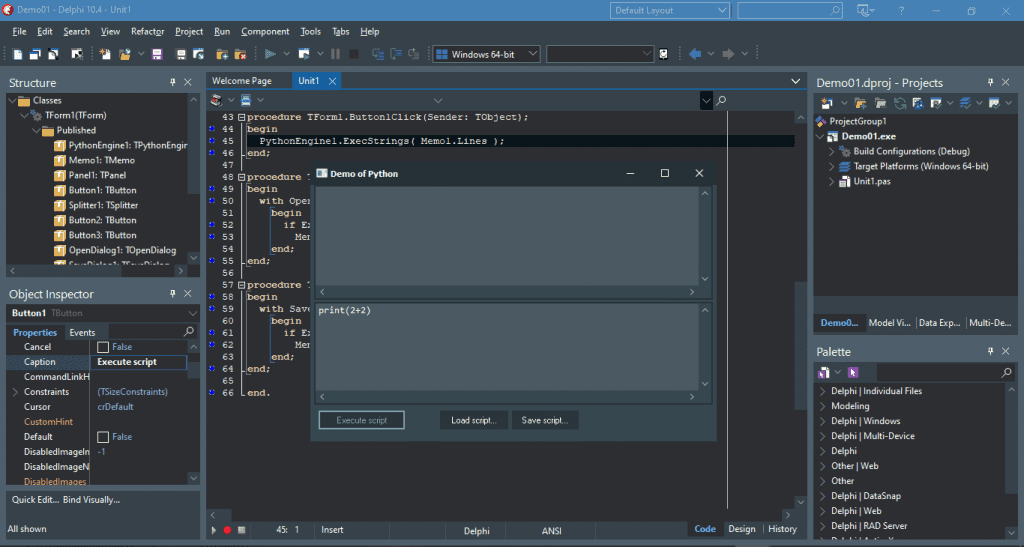Open Demo01.dproj

Python has two primitive loop commands:

• for loops
• while loops

Anyway, in this post, we will also study nested loops and loop control statements.

## 1. Python “for” Loop

“For” loop in Python is used to iterate over a sequence of items like list, tuple, set, dictionary, string, or any other iterable objects. This is less like the “for” keyword in other programming languages, and works more like an iterator method as found in other object-orientated programming languages. The “for” loop continues until we reach the end of the sequence.

Insert the following example to our Python GUI (lower Memo):

Click the Execute button, and let’s see the result in the upper Memo: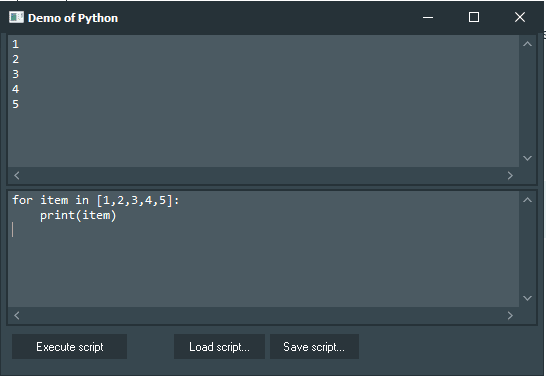Basic Example of Python for Loop using Python GUI from Python4Delphi.

### 1.1. The range() Function

When using for loops in Python, the range() function is pretty useful to specify the number of times the loop is executed. It yields a sequence of numbers within a specified range. Let’s see this with an example:

See the output in the upper Memo: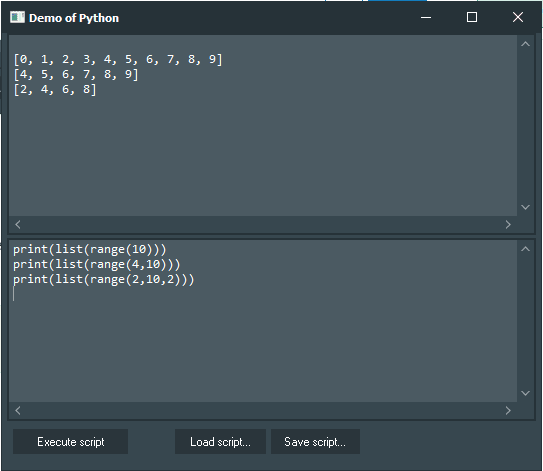Basic Example of range() Function using Python GUI from Python4Delphi.

### 1.2. Iterating over range Object

You can use the for loop to iterate over the range of objects. See the example below:

See the output in the upper Memo:Basic Example of Iterating over the range Object using Python GUI from Python4Delphi.

### 1.3. Using else in for Loop

In Python programming, the loops can also have an else part which will be executed once the loop terminates. See the example below:

See the output in the upper Memo:Basic Example of using else in for Loop using Python GUI from Python4Delphi.

## 2. Python “while” Loop

The while loop in Python executes a set of statements as long as a condition is True (until the specified condition becomes False).

Insert the following example to our Python GUI (lower Memo):

Click the Execute button, and let’s see the result in the upper Memo: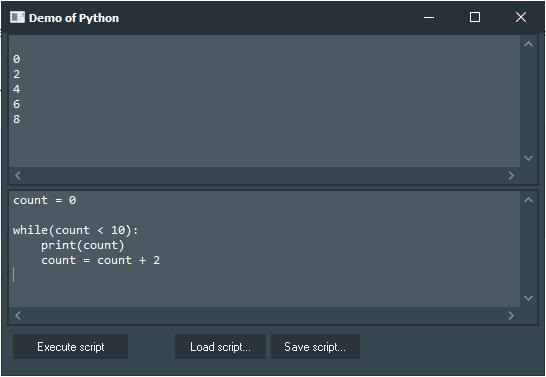Basic Example of Python while Loop using Python GUI from Python4Delphi.

### 2.1. Infinite Loop

A loop is called an infinite loop when the loop will never reach its end. Usually, when a condition is always True in a while loop, the loop will become an infinite loop. So we should be careful when writing conditions and while updating variables used in the loop. In Python shell or command prompt, we can terminate the program on an infinite loop by using CTRL + C.

Sometimes, we need to implement an infinite loop for example, when reading frames from a webcam.

Let’s see this with an example:

See the result in the upper Memo:Basic Example of Infinite Loop using Python GUI from Python4Delphi.

### 2.2. Using else in while Loop

The while loop may also have an else part after the loop. It is executed only when the condition of while loop becomes false. But if we break out of the loop before the condition has not reached false, then the else block does not get executed.

Let’s see this with an example:

See the result in the upper Memo: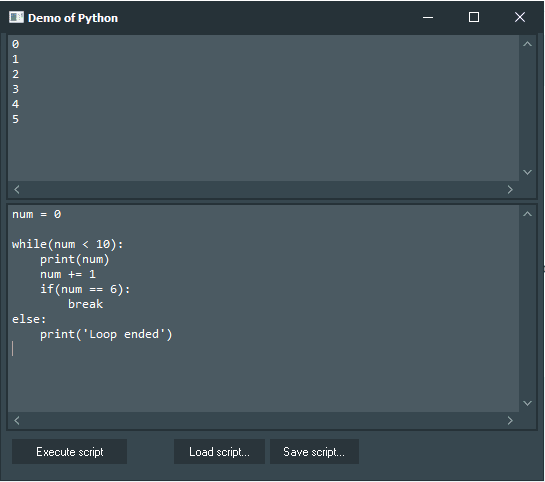Basic Example of Python else in while Loop using Python GUI from Python4Delphi.

## 3. Python Nested Loops

We can nest a loop inside another loop which simply means that a loop within a loop. Let’s see this with an example:

Click the Execute button, and let’s see the result in the upper Memo: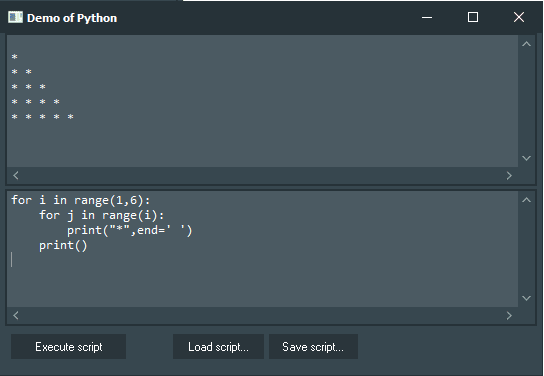Basic Example of Python Nested Loops using Python GUI from Python4Delphi.

From the example above, we can observe that the first iteration of the outer loop will run the whole inner loop and then in the next iteration of the outer loop, the inner loop gets executed again. This process is repeated until we reach the end of the outer loop.

## 4. Loop Control Statements in Python

Python allows us to control the flow of the execution of the program in a certain manner. For this we use the break, continue and pass keywords.

### 4.1. break

The break statement inside a loop is used to exit out of the loop. Sometimes in a program, we need to exit the loop when a certain condition is fulfilled.

Let’s see this with an example:

See the output in the upper Memo: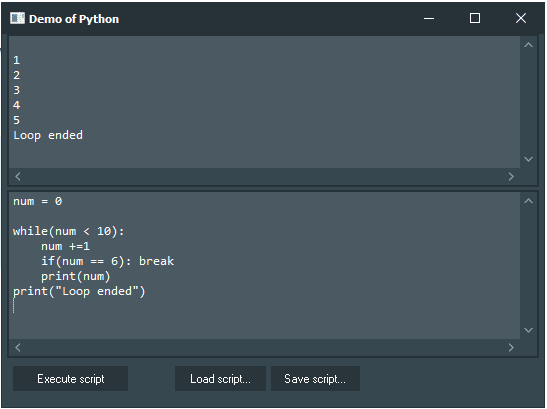Basic Example of Python break Statement using Python GUI from Python4Delphi.

### 4.2. continue

The continue statement is used to skip the next statements in the loop. When the program reaches the continue statement, the program skips the statements after continue and the flow reaches the next iteration of the loop.

Let’s see this with an example:

See the output in the upper Memo: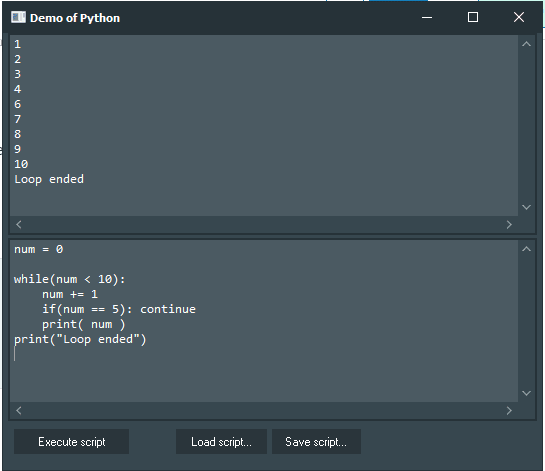Basic Example of Python continue Statement using Python GUI from Python4Delphi.

### 4.3. pass

The pass is a null statement and the Python interpreter returns a no-operation (NOP) after reading the pass statement. Nothing happens when the pass statement is executed. It is used as a placeholder when implementing new methods or we can use them in exception handling.

Let’s see this with an example:

See the output in the upper Memo: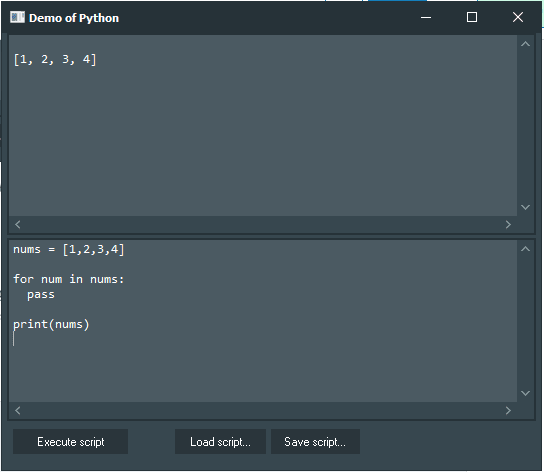Basic Example of Python pass Statement using Python GUI from Python4Delphi.

To sum up, we learned how the concepts of loops are useful for us programmers to do repetitive tasks effectively. We saw examples and syntax of different types of Python loops, which are for loop and while loop. We also saw that we can use else statements with a for or while loop. Moreover, we saw nested loop and loop control statements which helps to change the normal flow of the loop.

Check out Python4Delphi which easily allows you to build Python GUIs for Windows using Delphi.